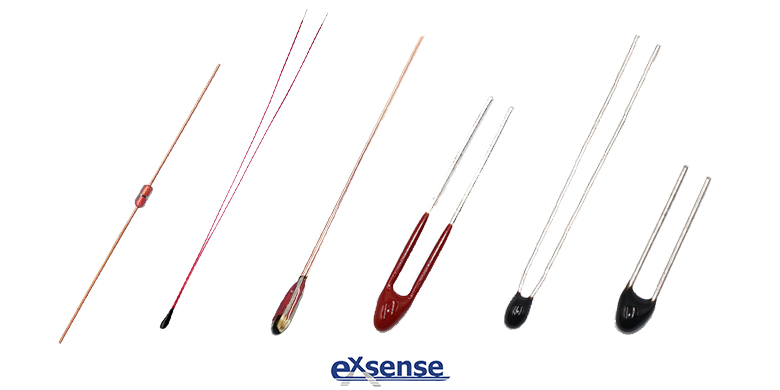QQCustomer Service
Product Catalogue
Tel :
+86-758-2661333
+86-15218448949
Fax :
+86-758-2698912
QQ :
1919442378/3060193679
No. 201, Shunjing Road, Dinghu, Zhaoqing
Technical Support
Technical Support
Analysis of NTC Thermistor
2021/02/16 03:02:38EXSENSE Electronics Technology Co., Ltd. is high-tech private enterprise which is specialized in research, production and sales of NTC thermistor, NTC chip and related materials, EXSENSE Electronics can provide customers with exclusive product customization, accurate product information and high-quality thermistor products.

NTC thermistor is a resistor that is sensitive to temperature. Its resistance value will change with the change of temperature. There is a linear relationship between ambient temperature and resistance value. The current temperature value can be determined by measuring the change of resistance value, this is the working principle of NTC thermistor for temperature measurement. The relationship between resistance value and temperature is shown in the figure below:In the process of temperature measurement, NTC thermistor is usually connected in series with a precise fixed value resistor, and the ambient temperature is determined by collecting voltage changes at both ends of the resistor. Common temperature measurement circuits are shown in the following figure:As shown in the figure above, in the circuit on the left, the NTC thermistor is in series with the fixed value resistor R. When the ambient temperature changes, the resistance value of the thermistor changes, resulting in changes of the voltage at its both ends. By collecting the voltage at both ends of the thermistor, the current temperature value can be deduced back, which is also one of the common ways of temperature measurement.

Due to the resistance value of NTC thermistor presents a certain linear relationship with temperature, and this linear relationship is related to B value (material constant of thermistor), therefore, the following formula of resistance value and temperature of NTC thermistor is generally used to obtain RT curve table:

Rt=Rn*EXP(B*(1/T-1/Tn))

Rt is the current resistance value of the thermistor;

Rn is the standard resistance value of the thermistor at Tn room temperature;

The B value is the temperature coefficient of the thermistor;

EXP is the nth power of e;

T and Tn refer to the K degrees i.e. Degree Kelvin, K degrees = 273.15 (absolute temperature) + degrees Celsius;

T is the current temperature;

Tn is 25℃ at room temperature.

NTC thermistor produced by EXSENSE Electronics Technology Co., Ltd. has characteristics of high precision (precision can up to ±0.5%, ±1%, ±2%, ±3%), great sensitivity and great reliability, which is widely used in cold chain transportation, smart home, optical communication, new energy automobile, aerospace and other fields.

mqu.cn site.nuo.cn